# CBSE Question Paper 2008 class 12 Chemistry

CBSE Question Paper 2008 class 12 Chemistry conducted by Central Board of Secondary Education, New Delhi in the month of March 2008. CBSE previous year question papers with the solution are available in the myCBSEguide mobile app and website. The Best CBSE App for students and teachers is myCBSEguide which provides complete study material and practice papers to CBSE schools in India and abroad.

CBSE Question Paper 2008 class 12 Chemistry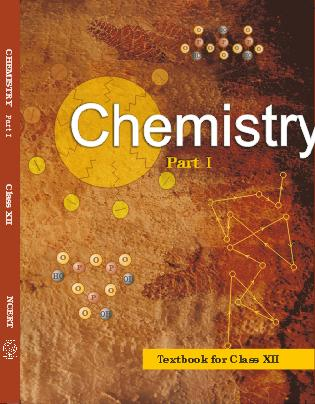## Class 12 Chemistry list of chapters

1. The Solid State
2. Solutions
3. Electrochemistry
4. Chemical Kinetics
5. Surface Chemistry
6. General Principles and Processes of Isolation of Elements
7. The p-Block Elements
8. The d and f Block Elements
9. Coordination Compounds
10. Haloalkanes and Haloarenes
11. Alcohols, Phenols and Ethers
12. Aldehydes, Ketones and Carboxylic Acids
13. Amines
14. Biomolecules
15. Polymers
16. Chemistry in Everyday life

## CBSE Question Paper 2008 class 12 Chemistry

### General Instructions

1. All questions are compulsory.
2. Marks for each question are indicated against it.
3. Question numbers 1 to 8 are very short-answer questions, carrying 1 mark each. Answer these in one word or about one sentence each.
4. Question numbers 9 to 18 are short-answer questions, carrying 2 marks each. Answer these in about 30 words each.
5. Question numbers 19 to 27 are short-answer questions of 3 marks each. Answer these in about 40 words each.
6. Question numbers 28 to 30 are long-answer questions of 5 marks each. Answer these in about 70 words each.
7. Use Log Tables, if necessary Use of calculators is not permitted.

1. What type of substances exhibits antiferromagnetism. 

2. Express the relation between conductivity and molar conductivity of a Solution. 

3. Which has a higher enthalpy of adsorption, physisorption of chemisorption? 

4. Write the IUPAC name of the following compound: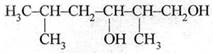5. In Which one of the two structures,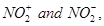the bond angle has a higher value? 

6. Name a substance that can be used as an antiseptic as well as a disinfectant. 

7. Arrange the following compounds in an increasing order of their acid Strengths.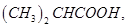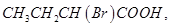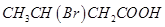8. Write a chemical reaction in which the iodide ion replaces the diazonium group in a diazonium salt. 

9. Formulate the galvanic cell in which the following reaction takes place: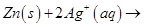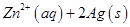State:
(i) Which one of its electrodes is negatively charged.
(ii) The reaction taking place at each of its electrode.
(iii) The carriers of current within this cell,

10. The resistance of a conductivity cell containing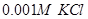Solution at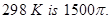What is the cell constant if the conductivity ofsolution at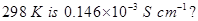11. State Raoult’s law for solutions of volatile liquids. Taking suitable examples explain the meaning of positive and negative deviations from Raoult’s law. 

Or

Define the term osmotic pressure. Describe how the molecular mass of a substance can be determined by a method based on measurement of Osmotic pressure.

12. Assign a reason for each of the following statements:  (i) Ammonia is a stronger base than phosphine.
(ii) Sulphur in vapour state exhibits a paramagnetic behavior.

13. Draw the structures of the following molecules:  (i)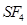(ii)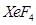14. Explain as to why haloarenes are much less reactive than haloalkanes towards nucleophilic substitution reactions. 

OR

Which compound in each of the following pairs will react faster in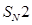reaction with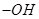? Why?
(i)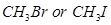(ii)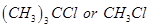15. (a) State the IUPAC name of the following compound: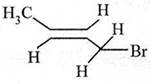(b) Complete the following chemical equation.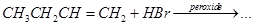16. What are biodegradable and non-biodegradable detergents. Give one example of each class. 

17. Define the following terms in relation to proteins: .
(ii) Denaturation

18. List the reactions of glucose which cannot be explained by its open chain structure. 

19. How Would you account for the following?  (i) Frenkel defects are not found in alkali metal halides.
(ii) Schottky defects lower the density of related solids.
(iii) Impurity doped Silicon is a semiconductor,

Or

Explain the following properties giving suitable examples:
(i) Ferromagnetism
(ii) Paramagnetism
(iii) Ferrimagnetism

20. Calculate the temperature at which a solution containing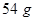of glucose,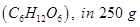of water will freeze.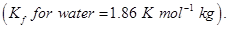21. Explain the basic principles of following metallurgical operations: 

(i) Zone refining
(ii) Vapour phase refining
(iii) Electrolytic refining

22. Explain What is observed when: 

(i) an electrolyte, KC, is added to hydrated ferric oxide solution
(ii) an electric current is passed through a colloidal Solution
(iii) a beam of strong light is passed through a colloidal solution

23. Write the names and structures of the monomers of the following polymers: 

(i) Buna-S
(ii) Neoprene
(iii) Nylon-6

24. Account for the following observations: 

(i)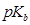for aniline is more than that for methylamine,
(ii) Methylamine solution in water reacts with ferric chloride solution to give a recipitate of ferric hydroxide,
(iii) Aniline does not undergo Friedel-Crafts reaction.

25. (a) What is a ligand? Give an example of a bidentate ligand.  (b) Explain as to how the two complexes of nickel,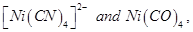have different structures but do not differ in their magnetic behaviour. (Ni = 28)

26. Name the reagents which are used in the following conversions: 

(i) A primary alcohol to an aldehyde
(ii) Butan-2-one to butan-2-ol
(iii) Phenol to 2, 4, 6-tribromophenol

27. Write chemical equations for the following processes:  (i) Chlorine reacts with a hot concentrated solution of sodium hydroxide
(ii) Orthophosphorous acid is heated
(iii)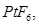and Xenon are mixed together

28. Assign reasons for the following: 

(i) The enthalpies of atomisation of transition elements are high,
(ii) The transition metals and many of their compounds act as good catalyst.
(iii). From element to element the actinoid contraction is greater than the lanthanoid contraction.
(iv) The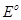value for the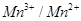couple is much more positive than that for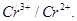(v). Scandium (Z = 21) does not exhibit variable oxidation states and yet it is regarded as a transition element.

OR

(a) What may be the possible oxidation states of the transition metals with the following d electronic configurations in the ground state of their atoms: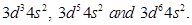Indicate relative stability of oxidation states in each case.

(b) Write steps involved in the preparation of
(i)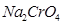from chromite ore and
(ii)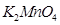from pyrolusite ore. [3, 2]

29. (a) Derive the general form of the expression for the half-life of a first Order reaction.
(b) The decomposition of NH on platinum surface is a zero-order reaction. What are the rates of production of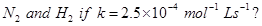[2, 3]

Or

(a) List the factors that affect the Tate of a chemical reaction.
(b) The half-life of radioactive decay of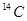is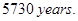An archaeological artefact containing wood had only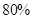ofactivity found in living tree. Estimate the age of the artefact. [2, 3]

30. (a) Complete the following reaction statements by giving the missing starting material, reagent or product as required: [3, 2]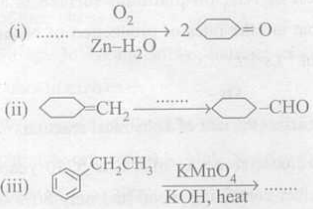(b) Describe the following reactions:
(i) Cannizaro reaction
(ii) Cross aldol condensation

OR

(a) How would you account for the following: [3, 2] (i) Aldehydes are more reactive than ketones towards nucleophiles.
(ii) The boiling points of aldehydes and ketones are lower than of the corresponding acids.
(iii) The aldehydes and ketones undergo a number of addition reactions.

(b) Give chemical tests to distinguish between:
(i) Acetaldehyde and benzaldehyde
(ii) Propanone and propanol

These are questions only. To view and download complete question paper with solution install myCBSEguide App from google play store or login to our student dashboard.

## Chemistry Question Paper 2008

Download class 12 Chemistry question paper with solution from best CBSE App the myCBSEguide. CBSE class 12 Chemistry question paper 2008 in PDF format with solution will help you to understand the latest question paper pattern and marking scheme of the CBSE board examination. You will get to know the difficulty level of the question paper. CBSE question papers 2008 for class 12 Chemistry have 30 questions with solution.

## Previous Year Question Paper for class 12 in PDF

CBSE question papers 2018, 2017, 2016, 2015, 2014, 2013, 2012, 2011, 2010, 209, 2008, 2007, 2006, 2005 and so on for all the subjects are available under this download link. Practicing real question paper certainly helps students to get confidence and improve performance in weak areas.

To download CBSE Question Paper 2008 class 12 Accountancy, Chemistry, Physics, History, Political Science, Economics, Geography, Computer Science, Home Science, Accountancy, Business Studies and Home Science; do check myCBSEguide app or website. myCBSEguide provides sample papers with solution, test papers for chapter-wise practice, NCERT solutions, NCERT Exemplar solutions, quick revision notes for ready reference, CBSE guess papers and CBSE important question papers. Sample Paper all are made available through the best app for CBSE students and myCBSEguide website.### Test Generator

Create question paper PDF and online tests with your own name & logo in minutes.### myCBSEguide

Question Bank, Mock Tests, Exam Papers, NCERT Solutions, Sample Papers, Notes